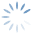Help OnLine | Chart – Spread ACF
3067

Click on the image to expand

It is called Autocorrelation and is constructed so that each histogram represents the correlation value obtained by evaluating the close of the 1500 sample bars using this procedure:

the first histogram is the average of the correlation calculated between the first bar and the second, between the second and third, between 1499 and 1500

the second histogram skips 1 and so it becomes the first with the third, second with fourth, 1498 with 1500the third skips 2 … 20 skips 19 and so becomes the first with 20 the second with 21, the third with 22 the 1481 with the 1500.

Observing the histograms I will have to note low values (less than 0.5) and opposite values (negative and positive).

In the image we have the autocorrelation of a perfect couple.

What should I observe:

since this graph is calculated on the Z-Score, we would like to have:

• As in the figure if we are waiting for the Z-Score to return to zero;
• With histograms that often oscillate between positive and negative if the Z-Score exceeds the two threshold values of 2 standard deviations, because since there is no longer a trend it is time to open the position.